Static Electricity - Lesson 3 - Electric Force

Newton's Laws and the Electrical ForceThe attractive or repulsive interaction between any two charged objects is an electric force. Like any force, its effect upon objects is described by Newton's laws of motion. The electric force - Felect - joins the long list of other forces that can act upon objects. Newton's laws are applied to analyze the motion (or lack of motion) of objects under the influence of such a force or combination of forces. The analysis usually begins with the construction of a free-body diagram in which the type and direction of the individual forces are represented by vector arrows and labeled according to type. The magnitudes of the forces are then added as vectors in order to determine the resultant sum, also known as the net force. The net force can then be used to determine the acceleration of the object.

In some instances, the goal of the analysis is not to determine the acceleration of the object. Instead, the free-body diagram is used to determine the spatial separation or charge of two objects that are at static equilibrium. In this case, the free-body diagram is combined with an understanding of vector principles in order to determine some unknown quantity in the midst of a puzzle involving geometry, trigonometry and Coulomb's law. In this last section of Lesson 3, we will explore both types of applications of Newton's laws to static electricity phenomenon.

Electric Force and Acceleration

Suppose that a rubber balloon and a plastic golf tube are both charged negatively by rubbing them with animal fur. Suppose that the balloon is tossed up into the air and the golf tube is held beneath it in an effort to levitate the balloon in midair. This goal would be accomplished when the spatial separation between charged objects is adjusted such that the downward gravity force (Fgrav) and the upward electric force (Felect) are balanced. This would present a difficult task of manipulation as the balloon would constantly move from side to side and up and down under the influences of both the gravity force and the electric force. When the golf tube is held too far below the balloon, the balloon would fall and accelerate downward. This would in turn decrease the separation distance and lead to an increase in the electric force. As the Felect increases, it would likely exceed the Fgrav and the balloon would suddenly accelerate upward. And finally, if the point of charge on the golf tube is not directly under the point of charge of the balloon (a likely scenario), the electric force would be exerted at an angle to the vertical and the balloon would have a sideways acceleration. The likely result of such an effort to levitate the balloon would be a variety of instantaneous accelerations in a variety of directions.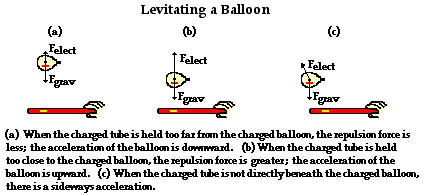Suppose that at some instant in the process of trying to levitate the balloon, the following conditions existed:

A 0.90-gram balloon with a charge of -75 nC is located a distance of 12 cm above a plastic golf tube that has a charge of -83 nC.

How could one apply Newton's laws to determine the acceleration of the balloon at this instant?

Like any problem involving force and acceleration, the problem would begin with the construction of a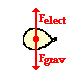free-body diagram. There are two forces acting upon the balloon. The force of gravity on the balloon is directed downward. The electric force on the balloon is exerted upward since the balloon and golf tube are like-charged and the golf tube is held below the balloon. These two forces are shown in the free-body diagram at the right. The second step involves determining the magnitude of these two forces. The force of gravity is determined by multiplying the mass (in kilograms) by the acceleration of gravity.

Fgrav = m • g = (0.00090 kg) • (9.8 m/s/s)

Fgrav = 8.82 x 10-3 N, down

The electric force is determined using Coulomb's law. As shown below, the appropriate unit on charge is the Coulomb (C) and the appropriate unit on distance is meters (m). Use of these units will result in a force unit of the Newton. The demand for these units emerges from the units on Coulomb's constant.

Felect = k • Q1 • Q2 /d2

Felect = (9 x 109 N•m2/C2) • (-75 x 10-9 C) • (-83 x 10-9 C) / (0.12)2

Felect = 3.89 x 10-3 N, up

The net force is the vector sum of these two forces. The upward and downward forces are added together as vectors.

Fnet = ·F = Fgrav (down) + Felect (up)

Fnet = 8.82 x 10-3 N, down + 3.89 x 10-3 N, up

Fnet = 4.93 x 10-3 N, down

The final step of this problem involves the use of Newton's second law to determine the acceleration of the object. The acceleration is the net force divided by the mass (in kilograms).

a = Fnet / m = (4.93 x 10-3 N, down) / (0.00090 kg)

a = 5.5 m/s/s, down

The above analysis illustrates how Newton's law and Coulomb's law can be applied to determine an instantaneous acceleration. The next analysis involves a case in which two objects are in a state of static equilibrium.

Electric Force and Static Equilibrium

Suppose that two rubber balloons are hung from the ceiling by two long strings such that they hang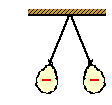vertically. Then suppose that each balloon is given 10 average-strength rubs with animal fur. The balloons, having a greater attraction for electrons than animal fur, would acquire a negative charge. The balloons would have the same type of charge and they would subsequently repel each other. The result of their repulsion is that the strings and suspended balloons would now make an angle with the vertical. The angle of the string with the vertical would be mathematically related to the quantity of charge on the balloons. As the balloons acquire a greater quantity of charge, the force of repulsion between them would increase and the angle that the string makes with the vertical would also increase. Like any situation involving electrostatic force, this situation can be analyzed using vector principles and Newton's laws.

Suppose that the following conditions existed.

Two 1.1-gram balloons are suspended from 2.0-meter long strings and hung from the ceiling. They are then rubbed ten times with animal fur to impart an identical charge Q to each balloon. The balloons repel each other and each string is observed to make an angle of 15 degrees with the vertical. Determine the electric force of repulsion, the charge on each balloon (assumed to be identical), and the quantity of electrons transferred to each balloon as a result of 10 rubs with animal fur.

Because of the complexity of the physical situation, it would be wise to represent it using a diagram. The diagram will serve as a means of identifying the known information for this situation. The diagram below depicts the two balloons with the string of length L and the angle "theta". The mass (m) of the balloons is known; it is expressed here in kilogram (the standard unit of mass). The distance between the balloons (a variable in Coulomb's law) is marked on the diagram and represented by the variable d. The vertical line extending from the pivot point on the ceiling is drawn; this vertical line is one side of a right triangle formed by the horizontal line connecting the balloons and the string extending from balloon to ceiling. This right triangle will be useful as we analyze the situation using vector principles. Note that the vertical line bisects the line segment connecting the balloons; thus, one side of the right triangle has a distance of d/2.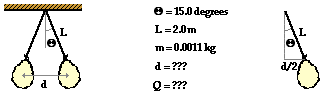The application of Newton's laws to this situation begins with the construction of a free-body diagram for one of the balloons. There are three forces acting upon the balloons: the tension force, the force of gravity and the electrostatic force of repulsion. These three forces are represented for the balloon on the right. (See diagram below.) Note that the tension force is directed at an angle to the vertical. In physics, such situations are treated by resolving the force vector into horizontal and vertical components. This is shown below; the components are labeled as Fx and Fy. These components are related to the angle that the string makes with the vertical by trigonometric functions. Since the balloon is at equilibrium, the forces that act upon the balloon must balance each other. This would mean that the vertical component of the tension force (Fy) must balance the downward force of gravity (Fgrav). And the horizontal component of the tension force (Fx) must balance the rightward electrostatic force (Felect).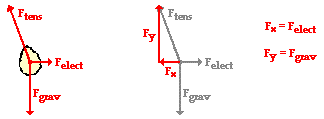Since the mass of the balloon is known, the force of gravity acting upon it can be determined.

Fgrav = m •g = (0.0011 kg) • (9.8 m/s/s)

Fgrav = 0.01078 N

The force of gravity is equal to the vertical component of the tension force (Fy = 0.0108 N ). The Fy component is related to the Fx component and the angle theta by the tangent function. This relationship can be used to determine the horizontal component of the tension force. The work is shown below.

Tangent(theta) = Fx / Fy

Tangent(15 degrees) = Fx / (0.01078 N)

Fx = (0.01078 N) • Tangent(15 degrees)

Fx = 0.00289 N

The horizontal component of the tension force is equal to the electrostatic force. Thus,

Felect = 0.00289 N

Now that the electrostatic force has been determined using Newton's laws and vector principles, Coulomb's law can now be applied to determine the charge on the balloon.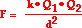It is assumed that the balloons have the same quantity of charge since they are charged in the same manner with 10 average-strength rubs. Since Q1 is equal to Q2, the equation can be rewritten as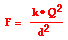This equation can be algebraically rearranged in order to solve for Q. The steps are shown below.

F • d2 = k • Q2

Q2 = F • d2 / k

Q = SQRT(F • d2 / k)

The value of d must be known to complete the solution. This demands that the right triangle be analyzed in order to determine the length of the side opposite the 15-degree angle. This length is one-half the distance d. Since the length of the hypotenuse is known, the sine function is used.

Sine(Theta) = opposite side / hypotenuse side

Sine(15 degrees) = opposite side / (2.0 m)

opposite side = (2.0 m) • Sine(15 degrees)

opposite side = d/2 = 0.518 m

Doubling this distance yields a value of d of 1.035 m. Now substitutions can be made in order to determine the value of Q.

Q = SQRT(F • d2 / k)

Q = SQRT [(0.00289 N) • (1.035 m)2 / (9 x 109 N•m2/C2)]

Q = 5.87 x 10-7 C (negative)

The charge on an object is related to the number of excess (or deficient) electrons in the object. Using the charge of a single electron (-1.6 x 10-19 C), the number of electrons on this object can be determined:

# excess electrons = (-5.87 x 10-7 C) / (-1.6 x 10-19 C/electron)

# excess electrons = 3.67 x 1012 electrons

During the charging process, more than three trillion electrons were transferred from the animal fur to each of the balloons. Wow!

Configurations of Three or More Charges

In each of the examples above, we have explored the interaction of two charged objects. Newton's laws and Coulomb's law were combined to analyze the situations. But what if there are three or more charges present? Coulomb's law can only consider the interaction between Q1 and Q2. Does the law for electric force have to be rewritten to account for a Q3? No!

Electrical forces result from mutual interactions between two charges. In situations involving three or more charges, the electric force on a single charge is merely the result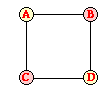of the combined effects of each individual charge interaction of that charge with all other charges. If a particular charge encounters two or more interactions, then the net electric force is the vector sum of those individual forces. As an example of this approach, suppose that four charges (A, B, C, and D) are present and that they are spatially arranged to form a square. Charges A and D are both negatively charged and occupy opposite corners of the square and Charges B and C are both positively charged and occupy the remaining two corners as shown. If one is concerned with the net electric force acting upon charge A, then the electric forces between A and each of the other three charges must be calculated. That is, FBA, FCA and FDA must first be determined by the application of Coulomb's law to each of these pairs of charges. The notation FBA is used to denote the force of B on A.

FBA = k • QA • QB / dBA2

FCA = k • QA • QC / dCA2

FDA = k • QA • QD / dDA2

The direction of each of these three forces can be determined by applying the basic rules of charge interaction: oppositely charged objects attract and like-charged objects repel. Applied to this scenario, one would reason that the forces FBA, FCA and FDA are directed as shown in the diagram below. Charge B attracts A and Charge C attracts A since these are pairs of oppositely charged objects. But Charge D repels A since they are a pair of like-charged objects.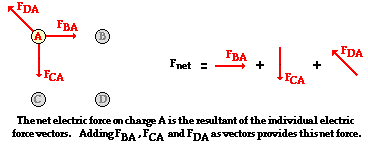So the magnitudes of the individual forces are determined through Coulomb's law calculations. The direction of the individual forces are determined by applying the rules of charge interactions. And once the magnitude and direction of the three force vectors are known, the three vectors can be added using rules of vector addition in order to determine the net electric force. This is illustrated in the diagram above.

Use your understanding of charge to answer the following questions. When finished, click the button to view the answers.

1. A positively charged object with a charge of +85 nC is being used to balance the downward force of gravity on a 1.8-gram balloon that has a charge of -63 nC. How high above the balloon must the object be held in order to balance the balloon? (NOTE: 1 nC = 1 x 10-9 C)

2. Balloon A and Balloon B are charged in a like manner by rubbing with animal fur. Each acquires an excess of 25 trillion electrons. If the mass of the balloons is 1 gram, then how far below Balloon B must Balloon A be held in order to levitate Balloon B? Assume the balloons act as point charges.

3. Two 1.2-gram balloons are suspended from light strings attached to the ceiling at the same point. The net charge on the balloons is -540 nC. The balloons are distanced 68.2 cm apart when at equilibrium. Determine the length of the string.

4. ZINGER: Three charges are placed along the X-axis. Charge A is a +18 nC charge placed at the origin. Charge B is a -27 nC charge placed at the 60 cm location. Where along the axis (at what x-coordinate?) must positively charged C be placed in order to be at equilibrium?